# nLab flavour anomaly

Contents

### Context

#### Fields and quanta

field (physics)

standard model of particle physics

force field gauge bosons

scalar bosons

flavors of fundamental fermions in the
standard model of particle physics:
generation of fermions1st generation2nd generation3d generation
quarks ($q$)
up-typeup quark ($u$)charm quark ($c$)top quark ($t$)
down-typedown quark ($d$)strange quark ($s$)bottom quark ($b$)
leptons
chargedelectronmuontauon
neutralelectron neutrinomuon neutrinotau neutrino
bound states:
mesonslight mesons:
pion ($u d$)
ρ-meson ($u d$)
ω-meson ($u d$)
f1-meson
a1-meson
strange-mesons:
ϕ-meson ($s \bar s$),
kaon, K*-meson ($u s$, $d s$)
eta-meson ($u u + d d + s s$)

charmed heavy mesons:
D-meson ($u c$, $d c$, $s c$)
J/ψ-meson ($c \bar c$)
bottom heavy mesons:
B-meson ($q b$)
ϒ-meson ($b \bar b$)
baryonsnucleons:
proton $(u u d)$
neutron $(u d d)$

(also: antiparticles)

effective particles

hadron (bound states of the above quarks)

solitons

minimally extended supersymmetric standard model

superpartners

bosinos:

dark matter candidates

Exotica

auxiliary fields

# Contents

## Idea

In the current standard model of particle physics the fundamental particles in the three generations of fermions have identical properties from one generation to the next, except for their mass, a state of affairs referred to as lepton flavour universality (LFU). A possible violation of lepton flavour universality is called a flavour anomaly, which would be a sign of “New Physics” (NP) beyond the standard model.

The presence – or not – of flavour anomalies is part of the general flavour problem of the standard model of particle physics.

Flavor universality pertains in particular to leptonic decays of bottom quarks, denoted $b$ (in the third generation), into electron/positron pairs, denoted $e^+ e^-$ (in the first generation) or muon/antimuon pairs, denoted $\mu^+ \mu^-$ (in the second generation), the latter two jointly denoted $\ell^+ \ell^-$ (for “leptons”) in this context.

Since quarks never appear in isolation (due to confinement) but always as bound states to hadrons, decays specifically of bottom quarks manifest themselves in leptonic decays of mesons that contain $b$B-mesons, denoted $B$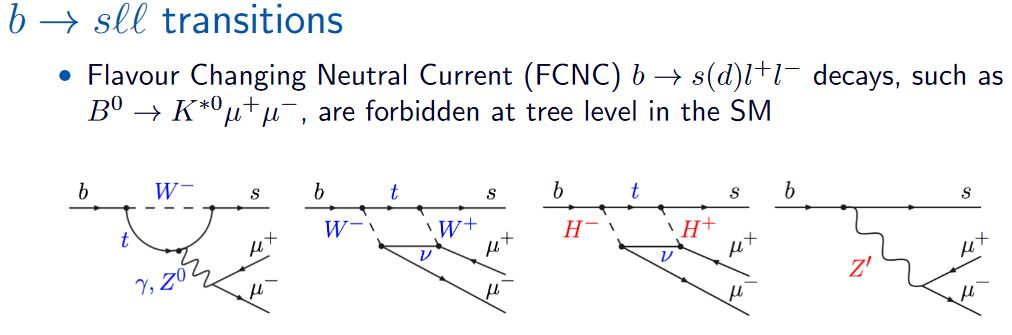graphics grabbed from Cartelle 18 – here $s$ denotes a strange quark

or as leptonic decays of baryons that contain $b$Lambda baryons, denoted $\Lambda_b$ (measured only more recently (LHCb 19120.8139)).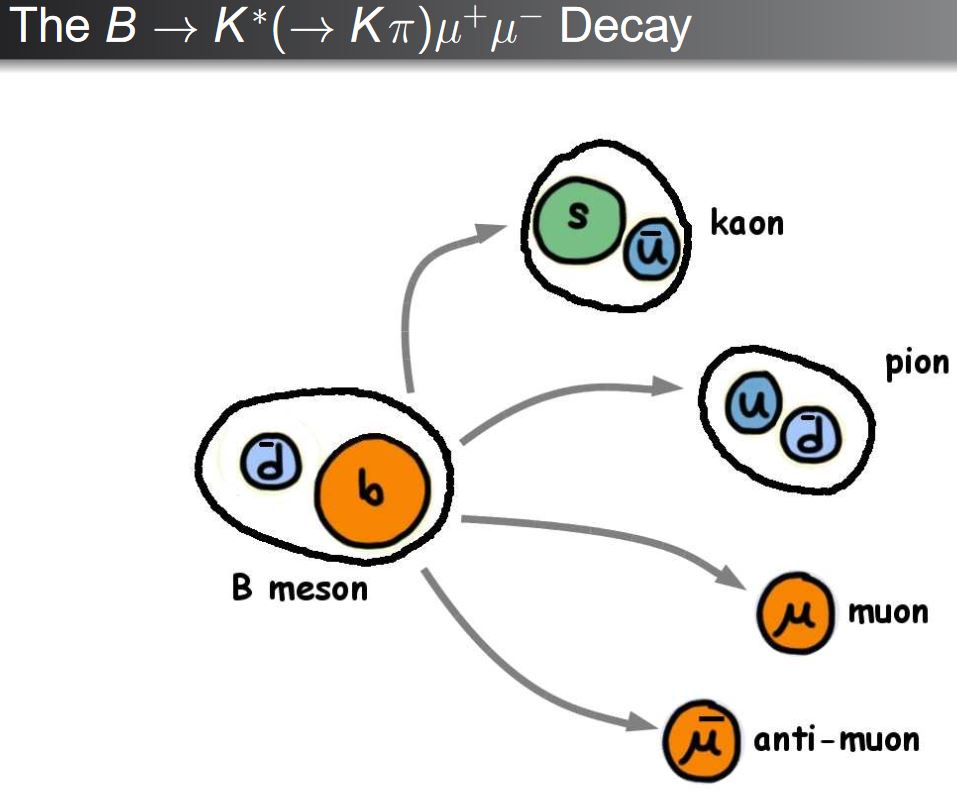Hence flavour universality in the standard model of particle physics demands, for example, that the ratios of decay rates (branching fractions) of B-mesons into electrons are the same as into muons, hence that the ratio

(1)$R_K \;=\; \frac{ B \to K \mu^+ \mu }{ B \to K e^+ e^- }$

(where $K$ is a kaon, a meson containing a strange quark)

is essentially equal to 1. Any significant departure of this observable from 1 would be a flavour anomaly and would mean that there are fundamental processes at play which are not described by the current standard model of particle physics (“New Physics”).

graphics from Altmannshofer 14

Moreover, in the standard model the decays involved here are “forbidden” (have vanishing decay rate) at tree level, so that only loop orders contribute to any possible flavour anomaly. But these loop contributions are sensitive to effects from putative undiscovered fundamental particles which “run in the loops” as virtual particles, and which thus may show up as a flavour anomaly even if their mass is too large for them to be seen as scattering products (i.e. as actual, non-virtual particles). This way tests of lepton flavour universality provide a means to potentially detect New Physics with indirect high-luminosity/high-precision experiments (see e.g. AHRS 20), complementary to the traditional high-energy direct detection measurements.

Notice that the channel $R_K$ (1) is just one of many in which flavour anomalies may and have been seen. There are other decay channels of B-mesons and there are also flavour anomalies in decays of, for instance, kaons (Buras 16, Buras 18).

See Alonso 19 for general introduction to an audience with basic background in quantum field theory.

## Statistical significance

Indications of flavour anomalies have been observed consistently and with increasing statistical significance around $2.5-3$ σ in B meson processes in various decay channels and by a number of independent experiments: the LHCb experiment, the Belle experiment and the BaBar experiment: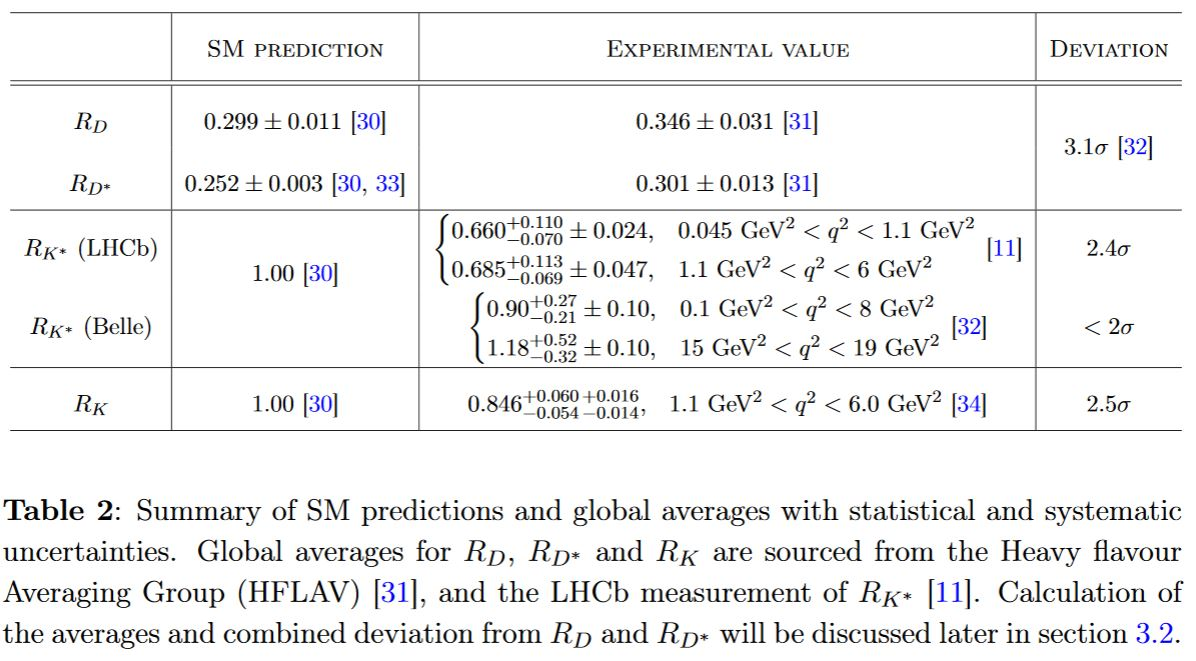table grabbed from BGV 19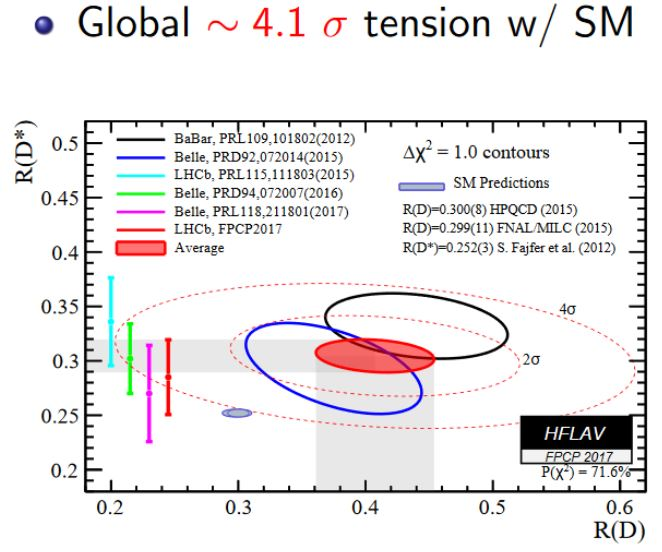The global (all channels and experiments combined) tension with the standard model by end of 2018 had statistical significance around $4.1$ σ (Strumia et al. 17, Cartelle 18, slide 22/25, Dey 18, slide 16/23, HAMN 18, p. 4, Bouchard-Cao-Owen 19). Various authors see the global tension (i.e. of all decay channels jointly) at over $5 \sigma$ (CCDGMV 17, CFMVV 17 CGMS 18, Dordei 18, slide 12), which traditionally qualifies as detection (see here), some authors already quote $5.3 \sigma - 5.8 \sigma$ (ACDGMM 19) or $5-6 \sigma$ (Kumar-London 19, Kumar 19).

graphics grabbed from Dey 18

This situation was confirmed with the completed measurements presented at Moriond 2019, which showed (Straub 19, Allanach 19) smaller mean discrepancy but also had smaller uncertainty, thus keeping the statistical significance of the apparent anomaly essentially unaffected. But Caria 19, slide 9 reported new measurements by Belle (Belle 1910) according to which the previous statistical significance of $3.8 \sigma$ in the $R_D$ channel would decrease to $3.1 \sigma$ or $2.8 \sigma$ (Gambino-Jung-Schacht 19 (6)). See LHCb 19, p. 2 for how the new numbers come about. The statistical significance over all sectors is still seen $\gt 4 \sigma$ (London 19, p. 7) or $\sim 3\sigma$ (Jaiswal-Nandi-Patra 20). Moreover, the theoretical prediction is being corrected further away from these measured values (BDKL 20, footnote 1).

Comprehensive assessments of the situation after Moriond 2019 are given in AHMSN 19, Descotes-Genon 19, Bardhan-Ghosh 19, Alguero 19 and agree that the flavour anomalies have been confirmed:

It appears the reason is that, given the amount of independent measurements (~180!) a smaller deviation because of new physics is easier to accommodate than a large one. Thus the new measurements actually fit better with new physics.

(Axel Maas, reporting from ALPS2019, tweet, 23 Apr 2019)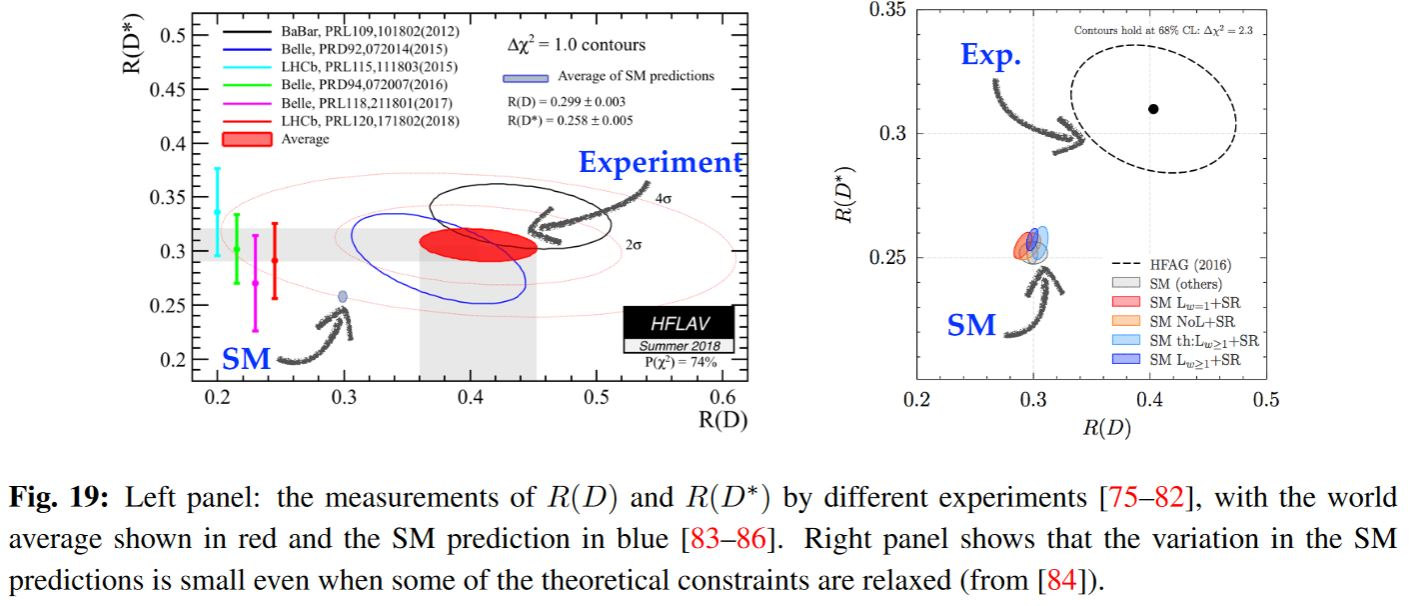Similarly Smith 20 on behalf of LHCb 2003.04831 (see also Lopes 20, CERN Courier 200311):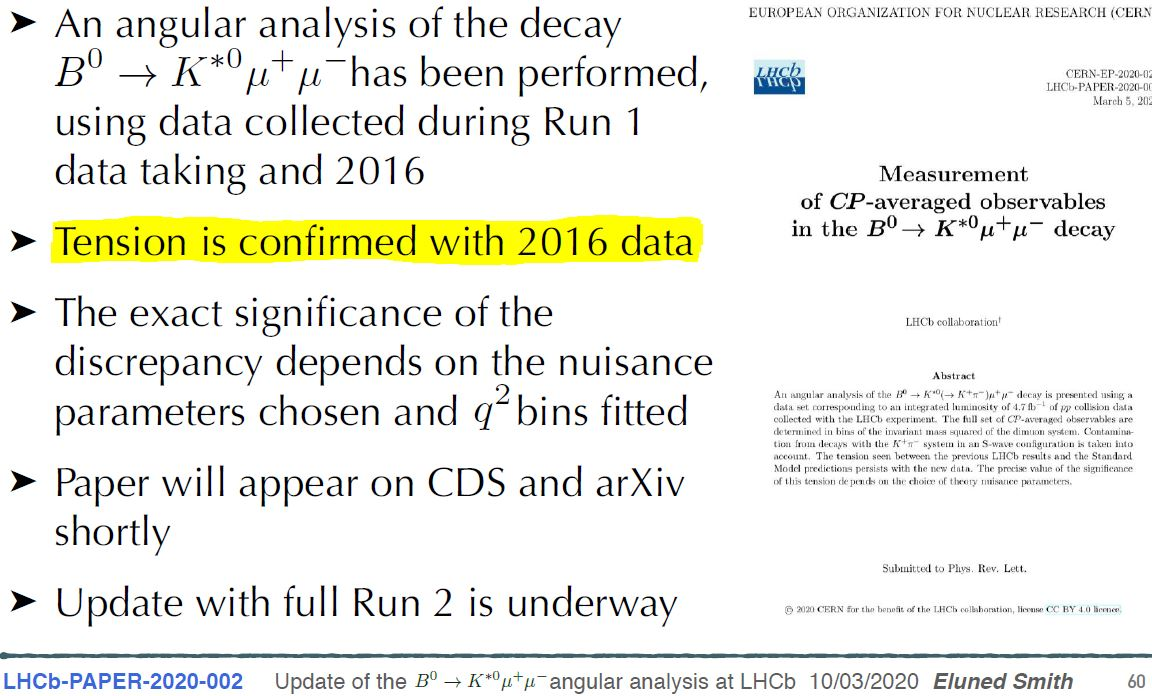The statistical significances of pull away from the standard model for effective New Physics models: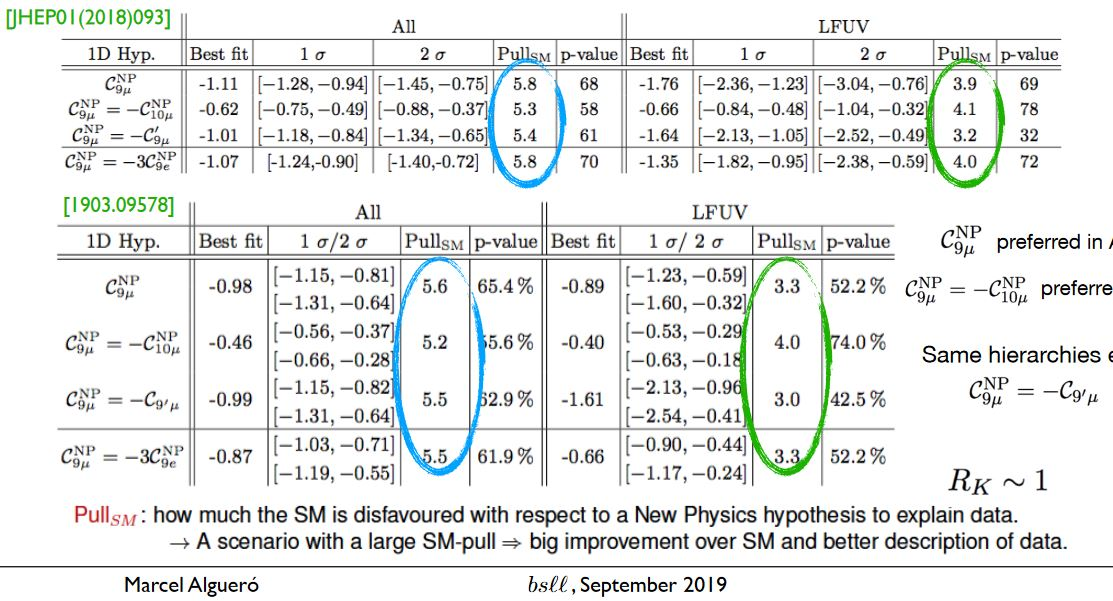table taken from Alguero 19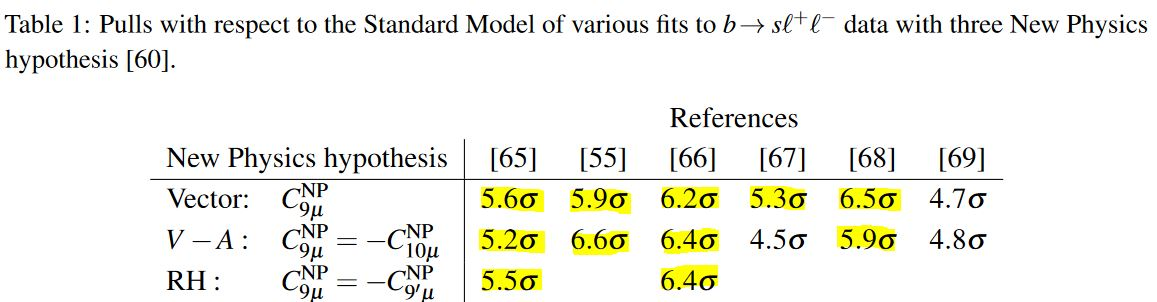table taken from Koppenburg 20

There is the claim (DGKV 19) that the discrepancy with the standard model increases further if non-trivial hadronic form factors are taken into account: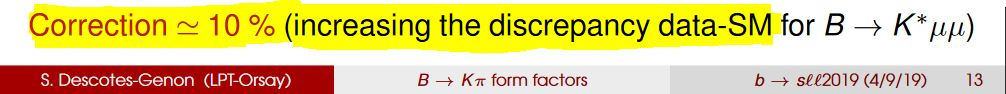slide grabbed from Descotes-Genon 19b

If the ongoing evaluation of the data of LHC‘s Run 2 confirms the measurements of Run 1, then the statistical significance of the effect in each decay channel separately should have reached 5 σ (Crivellin et. al 18, p. 12, Zupan 19, 4.6) and hence conventionally count as detection of flavour anomaly (see here), which would make it the first established “new physics” seen at the LHC. More recent extrapolation based on LHCb Collaboration 18 predicts a statistical significance between 6 and 10 $\sigma$ by the year 2025 (LKLR 19, p. 12).

In fact, it had been argued prior to the detection of the anomalies (Lyons 13b, Dorigo 15) that due to the specific nature of the experiment, the statistical significance-threshold for detection of anomalies in B meson-decays should not be taken to be $5 \sigma$, but just $3 \sigma$, hence well exceeded already by the experimentally seen statistical significance: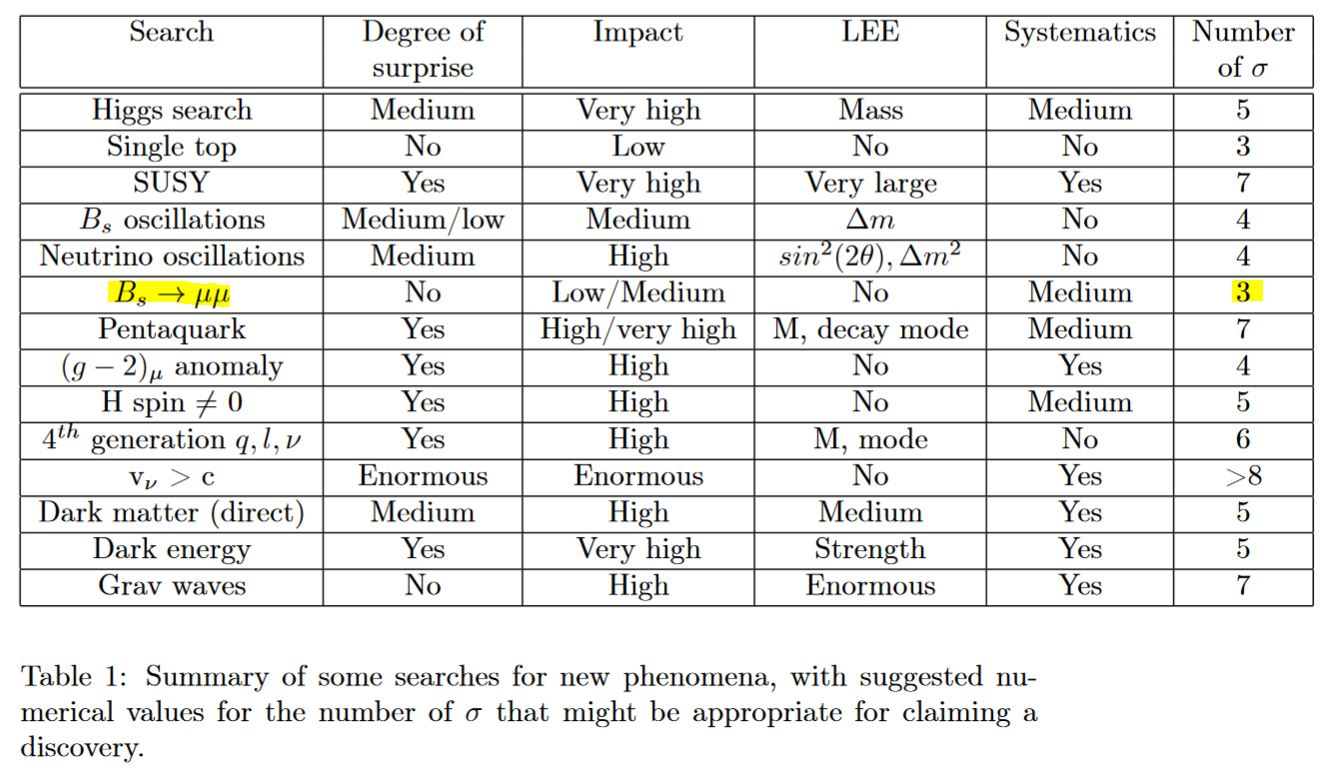table taken from Lyons 13b, p. 4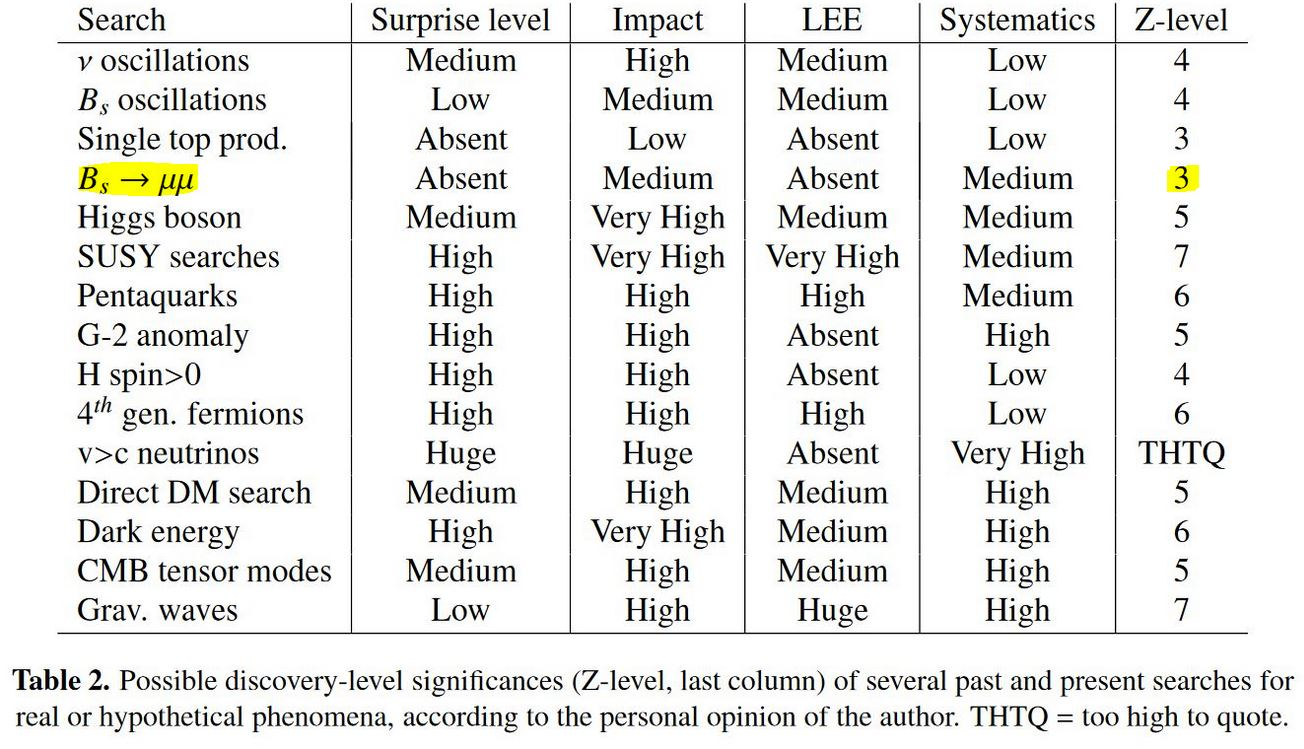table taken from Dorigo 15, p. 16

This attitude is reflected in SGGJC 19, p. 2:

For some time now, the ratios of semileptonic B-decay rates have appeared to be enhanced with respect to the Standard Model (SM) predictions with a global significance above the evidence threshold.

## Status and Outlook

In conclusion, with currently available data, the observed flavour anomalies are possible signs of New Physics beyond the current standard model of particle physics.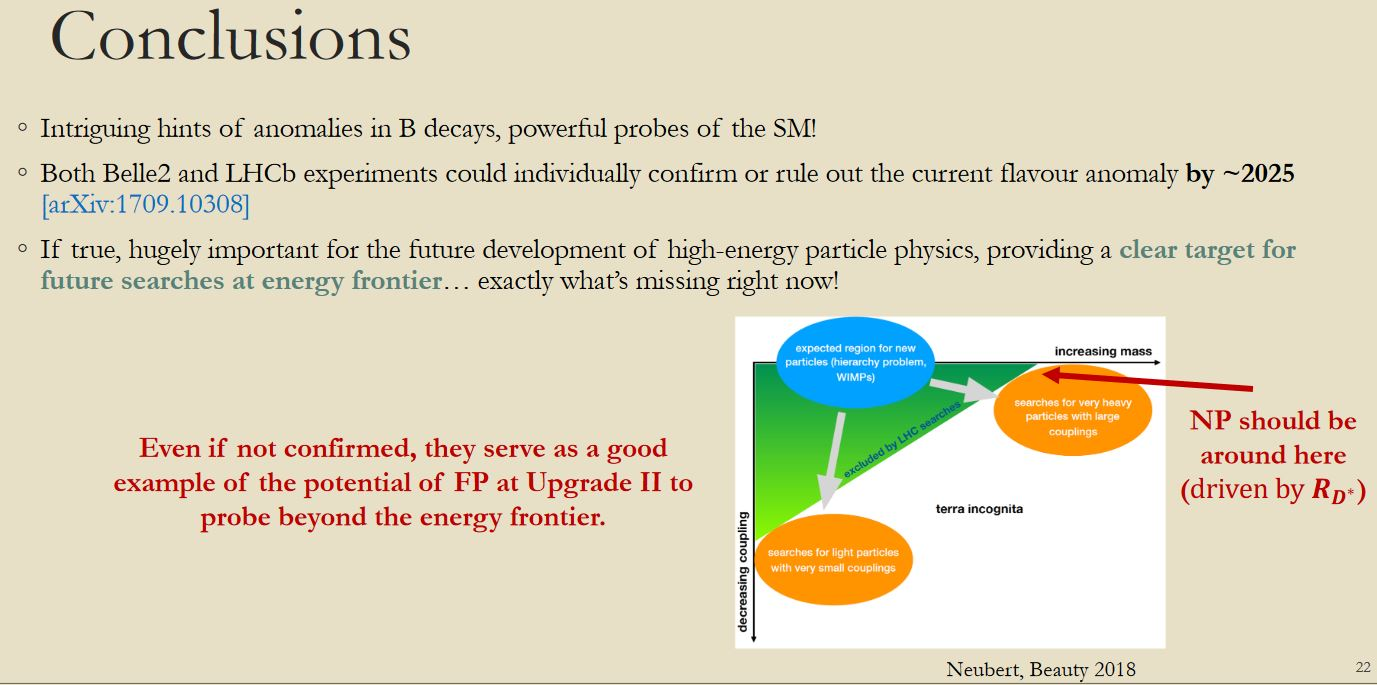graphics taken from Dordei 18, slide 22

While the statistical significance of the flavour anomalies in every single decay channel is about $2\sigma - 3 \sigma$, the effects are all compatible with each other, with a high joint/global statistical significance:

Garisto 20: Alone, each notable B physics result is only a few-sigma discrepancy. But taken together, the aggregate of the results is—depending on whom you ask—a 5- to 7-sigma deviation from the standard model estimates.

In any case, further and more sensitive experiments are needed to confirm and explore the effect, such as possibly the “HL-LHC” or “HE-LHC” experiment. General outlook, prospects and suggestions for future collider design in this respect are discussed in detail in Allanach-Gripaios-You 17, Crivellin et al. 18.

From Isidori 19, slide 6: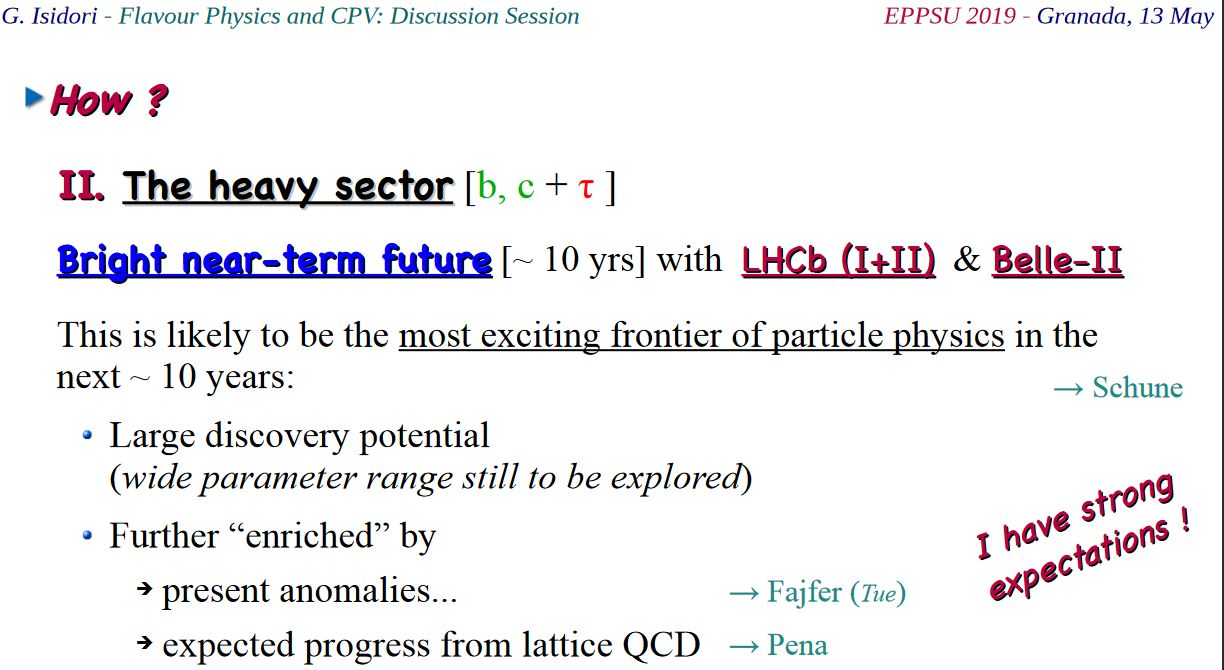Outlook on the Belle II experiement to confirm the flavour anomalies, if real (HHJLQV 20):

With $[$$]$ Belle II, we are at the brink of a new era in quark flavour physics $[...]$ we present a phenomenological study of the potential for Belle II to reveal possible new physics in the inclusive decay channel

(p. 15:) We see that anomalies in the exclusive sector can be confirmed at the level by inclusive measurements if the true values of $C_{9,10}^{\mu NP}$ are at the current best-fit point of the exclusive fits

(p. 16:) After including $B_s \to \mu^+ \mu_-$, the reach in the $[C_9^{\mu NP}, C_{10}^{\mu NP}]$ plane improves considerably. Exclusive anomalies could be confirmed at the level.

(p. 20:) Should the true value of $C_9^{NP}$ and $C_{10}^{NP}$ be at the current best-fit points of the global fits, an analysis of inclusive $\bar B_ \to X_s \ell^+ \ell^-$ at Belle II with $50 ab^{-1}$ of data will exclude the SM point $C_9^{NP} = C_{10}^{NP} = 0$ at the level of $\sim 5$σ. This again underlines the necessity of a full angular analysis of $\bar B_ \to X_s \ell^+ \ell^-$ at Belle II.

## Relation to other anomalies

There is possibly a relation between the flavour anomalies and the anomalies observed in the anomalous magnetic moment of the electron and/or the muon (e.g. Chiang-Okada 17) which might point to a common origin of these anomalies in the flavour sector (e.g. Crivellin-Hoferichter 20):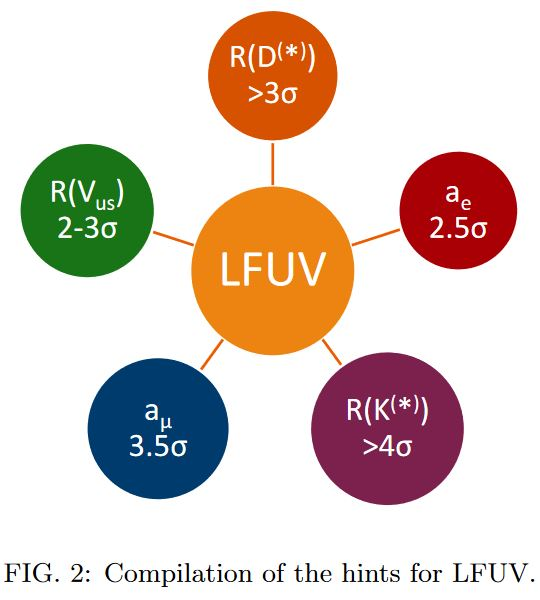graphics from Crivellin-Hoferichter 20

(here “$R$” refers to flavour anomalies in various channels, “$a$” refers to anomalies in the the anomalous magnetic moments of the electron and the muon, “LFUV” is shoft for “Lepton Flavor Universality Violation”, and the numbers are the statistical significances of the effects seen)

Indeed, leptoquark models preferred by the flavour anomalies (see below) are also a candidate explanation of the anomaly seen at over 4$\sigma$ statistical significance in the anomalous magnetic moment of the muon, see there.

## Possible models

Candidate models of “New Physics” beyond the standard model of particle physics that could possibly explain the flavour anomalies (if indeed they are real) includes the following:

### Leptoquarks and Grand unified theory

One promising model that could potentially explain the apparently observed flavour anomalies are leptoquarks, which naturally arise in, and hence potentially point to, grand unified theory models, such as notably the Pati-Salam model (Heek-Teresi 18, Heek-Teresi 19)

From Crivellin 18, p. 2:

the global fit $[$ to flavour anomalies $]$ even shows compelling evidence for New Physics $[$$]$ The vector leptoquark (LQ) $SU(2)_L$ singlet with hypercharge $-4/3$ arising in the famous Pati-Salam model is capable of explaining all the $[$flavour $]$ anomalies and therefore several attempts to construct a UV completion for this LQ to address the anomalies have been made. It can give a sizeable effect in $b \to c(u)\tau \nu$ data without violating bounds from $b \to s(d)\nu \bar \nu$ and/or direct searches, provides (at tree level) a $C_9 = - C_{10}$ solution to $b \to s \ell^+ \ell^-$ data and does not lead to proton decay at any order in perturbation theory.

From GMV 20, Figure 9, showing fits of leptoquark models (labeled $S_i$) to a variety of observables: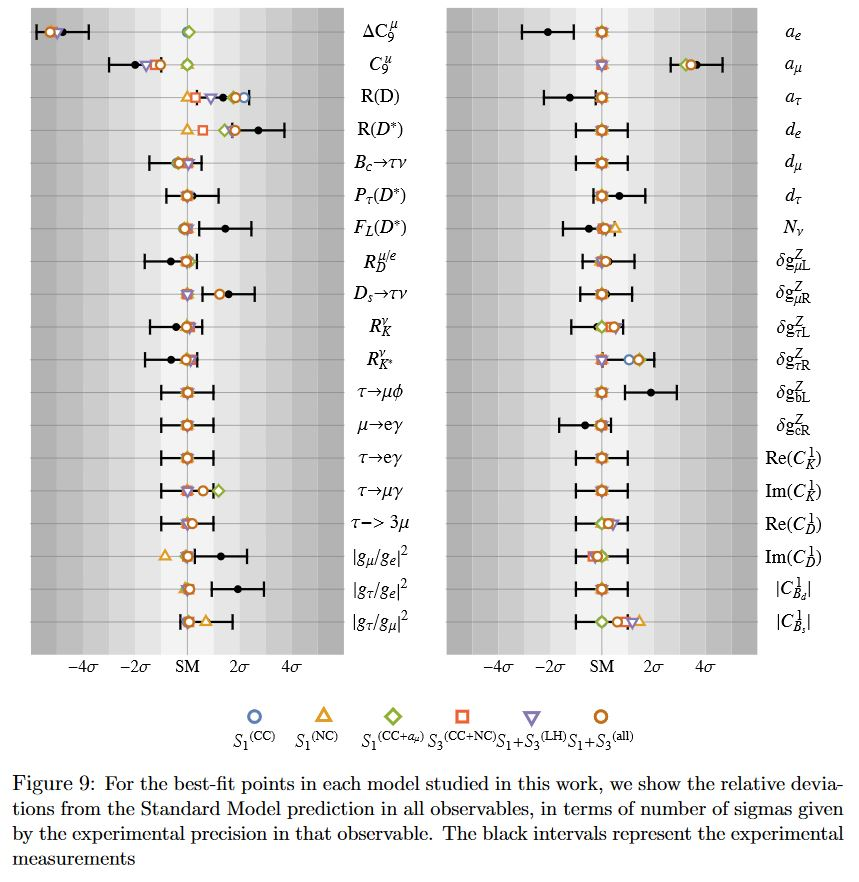### Other

Other possible models besides leptoquarks (above) which have been proposed as possible explanations of the apparently observed flavour anomalies include the following:

### General

Early suggestion to look at the $R_K$ channel at LHCb are due to

• Patrick Koppenburg, Selection of $B_u \to \ell \ell K$ at LHCb and Sensitivity to $R_K$, 2007 (cern:1027442, pdf)

General introduction to the issue is in

• Simone Bifani, Sebastien Descotes-Genon, Antonio Romero Vidal, Marie-Helene Schune, Review of Lepton Universality tests in B decays, Journal of Physics G: Nuclear and Particle Physics, Volume 46, Number 2 (2018) (arXiv:1809.06229)

• Rodrigo Alonso, Lepton (non-) unversality in (flavor changing) neutral current B decays, Proceedings of FPCP2019 (arXiv:1907.01716)

and for charm quark physics in

Comprehensive overview of the latest world-averaged results to be found at:

### Experimental results

• LHCb collaboration, Differential branching fractions and isospin asymmetries of $B \to K^{(\ast)}\mu^+ \mu^-$ decays, JHEP 06 (2014) 133 (arXiv:1403.8044)

• LHCb collaboration, Test of lepton universality using $B^+\to K^+ \ell^+ \ell^-$ decays, Phys. Rev. Lett. 113, 151601 (2014) (arXiv:1406.6482)

• LHCb collaboration, Angular analysis and differential branching fraction of the decay $B^0_s \to \phi \mu^+ \mu^-$, JHEP09(2015)179 (arXivL1506.08777)

• LHCb collaboration, Differential branching fraction and angular analysis of $\Lambda^0_b \to \Lambda \mu^+ \mu^-$ decays, JHEP 06 (2015) 115; JHEP 09 (2018) 145 (arXiv:1503.07138)

• LHCb collaboration, Test of lepton universality using $B^0\to K^{\ast 0} \ell^+ \ell^-$ decays, JHEP 08 (2017) 055 (arXiv:1705.05802)

• LHCb collaboration, Measurement of the ratio of the $B^0 \to D^{\ast -} \tau^+ \nu_\tau$ and $B^0 \to D^{\ast - }\mu^+ \nu_\mu$ branching fractions using three-prong τ-lepton decays (arXiv:1708.08856)

• Belle collaboration, Measurement of the $\tau$ lepton polarization and $R(D^\ast)$ in the decay $\bar B \to D^\ast \tau^- \bar \nu_\tau$ with one-prong hadronic τ decays at Belle (arXiv:1709.00129)

• Belle collaboration, Measurement of the $D^{\ast -}$ polarization in the decay $B^0 \to D^{\ast -} \tau^+ \nu_\tau$ (arXiv:1903.03102)

• LHCb collaboration, Search for lepton-universality violation in $B^+ \to K^+ \ell^+ \ell^-$ decays (cern:2668514)

• Belle Collaboration, Test of lepton flavor universality in $B \to K^\ast \ell^+ \ell^-$ decays at Belle (arXiv:1904.02440)

• LHCb collaboration, Lepton flavour universality in charged-current $B$ decays (arXiv:1907.01500)

• Belle collaboration, Measurement of $\mathcal{R}(D)$ and $\mathcal{R}(D^\ast)$ with a semileptonic tagging method, Phys. Rev. Lett. 124, 161803 (2020)

• LHCb collaboration, Test of lepton universality with $\Lambda^0_b\to p K^-\ell^+\ell^-$ decays (arXiv:1912.08139)

also:

CERN courier, Debut for baryons in flavour puzzle, 20 Dec. 2019

CERN news, LHCb explores the beauty of lepton universality, 15 Jan 2020

(first measurement of lepton universality for $\Lambda^0_b$ Lambda baryons, finding the flavour anomaly at (only) 1 sigma but pointing in the same direction as seen in other channels)

• LHCb collaboration, Measurement of CP-averaged observables in the $B^0 \to K^{\ast 0} \mu^+ \mu^-$ decay, Phys. Rev. Lett. 125, 011802 (cds:2712641, arXiv:2003.04831, doi:10.1103/PhysRevLett.125.011802)

• LHCb collaboration, Measurement of CP-averaged observables in the $B^0\to K^{\ast 0} \mu^+ \mu^-$ decay (spire:1784890, arXiv:2003.04831)

### Review and outlook

Outlook:

Outlook for Belle II to confirm the flavour anomalies, if they are real:

• Tobias Huber, Tobias Hurth, Jack Jenkins, Enrico Lunghi, Qin Qin, K.Keri Vos, Phenomenology of inclusive $\bar B \to X_s \ell^+ \ell^-$ for the Belle II era (arXiv:2007.04191)

Emphasis of non-perturbative effects:

• Ulrich Nierste, Flavour Anomalies: Phenomenology and BSM Interpretations, talk at Planck 2018, Bonn 2018 (pdf)

• Saeed Kamali, New physics in inclusive semileptonic $B$ decays including nonperturbative corrections (arXiv:1811.07393)

Emphasis of higher loop order-effects:

• Andreas Crivellin, Christoph Greub, Dario Müller, Francesco Saturnino, Importance of Loop Effects in Explaining the Accumulated Evidence for New Physics in B Decays with a Vector Leptoquark, Phys. Rev. Lett. 122, 011805 (2019) (arXiv:1807.02068)

Emphasis of effects of hadronic form factors:

• Sébastien Descotes-Genon, Alexander Khodjamirian, Javier Virto, Light-Cone Sum Rules for $B \to K \pi$ Form Factors and Applications to Rare Decays (arXiv:1908.02267)

• Sebastien Descotes-Genon, Light-cone sum rules for $B \to K \pi$ form factorsand applications to rare decays, talk at bsll2019 (pdf, pdf)

Original articles include:

• Guido D’Amico, Marco Nardecchia, Paolo Panci, Francesco Sannino, Alessandro Strumia, Riccardo Torre, Alfredo Urbano,

Flavour anomalies after the $R_{K^\ast}$ measurement,

J. High Energ. Phys. (2017) 2017 (arXiv:1704.05438)

• Andrea Mauri, Nicola Serra, Rafael Silva Coutinho, Towards establishing Lepton Flavour Universality violation in $\bar B \to \bar K^\ast \ell^+ \ell^-$ decays (arXiv:1805.06401)

• Bernat Capdevila, Andreas Crivellin, Sébastien Descotes-Genon, Joaquim Matias, Javier Virto, Patterns of New Physics in $b \to s \ell^+ \ell^-$ transitions in the light of recent data, JHEP 1801 (2018) 093 (arXiv:1704.05340)

• Alejandro Celis, Javier Fuentes-Martin, Avelino Vicente, Javier Virto, Gauge-invariant implications of the LHCb measurements on Lepton-Flavour Non-Universality, Phys. Rev. D 96, 035026 (2017) (arXiv:1704.05672)

• Monika Blanke, Andreas Crivellin, Stefan de Boer, Teppei Kitahara, Marta Moscati, Ulrich Nierste, Ivan Nišandžić, Impact of polarization observables and $B_c \to \tau \nu$ on new physics explanations of the $b \to c \tau \nu$ anomaly (arXiv:1811.09603)

• Monika Blanke, Andreas Crivellin, Stefan de Boer, Teppei Kitahara, Marta Moscati, Ulrich Nierste, Ivan Nišandžić, Addendum: “Impact of polarization observables and $B_c \to \tau \nu$ on new physics explanations of the $b \to c \tau \nu$ anomaly” (arXiv:1905.08253)

• Jacky Kumar, David London, New physics in $b \to s e^+ e^-$?, Phys. Rev. D 99, 073008 (2019) (arXiv:1901.04516, doi:10.1103/PhysRevD.99.073008)

• Domagoj Leljak, Blazenka Melic, Monalisa Patra, On lepton flavour universality in semileptonic $B_c \to \eta_c, J/\psi$ decays (arXiv:1901.08368)

• Rui-Xiang Shi, Li-Sheng Geng, Benjamín Grinstein, Sebastian Jäger, Jorge Martin Camalich, Revisiting the new-physics interpretation of the $b \to c \tau \nu$ data (arXiv:1905.08498)

Cautionary remarks include

In contrast, an argument that the threshold statistical significance for flavour anomalies should be taken to be $3\sigma$ instead of $5 \sigma$ is made in

Discussion of possible $b \to d$ anomalies:

• Aleksey V. Rusov, Probing New Physics in $b \to d$ Transitions (arXiv:1911.12819)

• Marzia Bordone, Nico Gubernari, Tobias Huber, Martin Jung, Danny van Dyk, A puzzle in B¯0(s)→D+(s){π−,K−} decays and extraction of the fs/fd fragmentation fraction (arXiv:2007.10338)

• Syuhei Iguro, Teppei Kitahara, Implications for new physics from novel puzzle in B¯0(s)→D(∗)+(s){π−,K−} decays (arXiv:2008.01086)

• Eleftheria Malami, Exploring New Physics in B→πK Decays (arXiv:2008.08468)

### Relation to $(g-2)$-anomalies

Beyond their possible common origin in leptoquarks, further possible joint explanation of flavour anomalies and the anomalies observed in the muon anomalous magnetic moment:

• Cheng-Wei Chiang, Hiroshi Okada, A simple model for explaining muon-related anomalies and dark matter (arXiv:1711.07365)

• Junichiro Kawamura, Stuart Raby, Andreas Trautner, Complete Vector-like Fourth Family and new $U(1)'$ for Muon Anomalies (arXiv:1906.11297)

• Lorenzo Calibbi, M.L. López-Ibáñez, Aurora Melis, Oscar Vives, Muon and electron $g-2$ and lepton masses in flavor models (arXiv:2003.06633)

• A. S. de Jesus, S. Kovalenko, F. S. Queiroz, K. Sinha, C. Siqueira, Vector-Like Leptons and Inert Scalar Triplet: Lepton Flavor Violation, $g-2$ and Collider Searches (arXiv:2004.01200)

• Shaikh Saad, Combined explanations of $(g-2)_\mu$, $R_{D^\ast}$, $R_{K^\ast}$ anomalies in a two-loop radiative neutrino mass model (arXiv:2005.04352)

• K. S. Babu, P. S. Bhupal Dev, Sudip Jana, Anil Thapa, Unified Framework for $B$-Anomalies, Muon $g-2$, and Neutrino Masses (arXiv:2009.01771)

### Possible explanations/models

#### General EFT parameterization

General effective field theory parametrization:

• T. Hurth, A. Arbey, F. Mahmoudi, S. Neshatpour, New global fits to $b \to s$ data with all relevant parameters, Proceedings of the Seventh Workshop on Theory, Phenomenology and Experiments in Flavour Physics, Capri, 8-10 June 2018 (arXiv:1812.07602)

• Srimoy Bhattacharya, Aritra Biswas, Zaineb Calcuttawala, Sunando Kumar Patra, An in-depth analysis of $b \to c(s)$ semileptonic observables with possible $\mu \to \tau$ mixing (arXiv:1902.02796)

• Marcel Algueró, Bernat Capdevila, Sébastien Descotes-Genon, Pere Masjuan, Joaquim Matias, What $R_K$ and $Q_5$ can tell us about New Physics in $b \to s \ell \ell$ transitions? (arXiv:1902.04900)

• Marcel Algueró, Bernat Capdevila, Andreas Crivellin, Sébastien Descotes-Genon, Pere Masjuan, Joaquim Matias, Javier Virto, Addendum: “Patterns of New Physics in $b \to s \ell^+ \ell^-$ transitions in the light of recent data” (arXiv:1903.09578)

• Ashutosh Kumar Alok, Amol Dighe, Shireen Gangal, Dinesh Kumar, Continuing search for new physics in $b \to \mu s s$ decays: two operators at a time (arXiv:1903.09617)

• Marco Ciuchini, António M. Coutinho, Marco Fedele, Enrico Franco, Ayan Paul, Luca Silvestrini, Mauro Valli, New Physics in $b \to s \ell^+ \ell^-$ confronts new data on Lepton Universality (arXiv:1903.09632)

• Jason Aebischer, Wolfgang Altmannshofer, Diego Guadagnoli, Meril Reboud, Peter Stangl, David M. Straub, B-decay discrepancies after Moriond 2019 (arXiv:1903.10434)

• Alakabha Datta, Jacky Kumar, David London, The $B$ Anomalies and New Physics in $b \to s e^+ e^-$ (arXiv:1903.10086)

• Ashutosh Kumar Alok, Dinesh Kumar, Suman Kumbhakar, S Uma Sankar, Impact of $D^\ast$ polarization measurement on solutions to $R_D$-$R_{D^\ast}$ anomalies (arXiv:1903.10486)

• Pere Arnan, Andreas Crivellin, Marco Fedele, Federico Mescia, Generic Loop Effects of New Scalars and Fermions in $b \to s \ell^+ \ell^-$ and a Vector-like 4th Generation (arXiv:1904.05890)

• A. Arbey, T. Hurth, F. Mahmoudi, D. Martinez Santos, S. Neshatpour, Update on the $b \to s$ anomalies (arXiv:1904.08399)

• Pouya Asadi, David Shih, Maximizing the Impact of New Physics in $b \to c \tau \nu$ Anomalies, (arXiv:1905.03311)

• J. E. Chavez-Saab, Marxil Sánchez, Genaro Toledo, $R_{D^\ast}$ or $R_{D_\pi}$: closing the theoretical gap? (arXiv:1905.03394)

• Suman Kumbhakar, Ashutosh Kumar Alok, Dinesh Kumar, S Uma Sankar, Resolving $R_D$ and $R_{D^\ast}$ anomalies (arXiv:1905.03513)

• Jyoti Saini, Dinesh Kumar, Shireen Gangal, S. B. Dasm, Probing signatures of beyond standard model physics through $B_s^\ast \to \mu^+ \mu^-$ decay (arXiv:1905.03933)

• David London, CP Violation in $\bar B^0 \to D^{\ast +} \ell^- \bar \nu_\ell$ (arXiv:1906.07752)

• Marta Moscati, New Physics in $b \to c \tau \nu$: Impact of Polarisation Observables and $B_c \to \tau \nu$ (arXiv:1906.08035)

• Damir Becirevic, Marco Fedele, Ivan Nisandzic, Andrey Tayduganov, Lepton Flavor Universality tests through angular observables of $\bar B \to D^{(\ast) \ell \bar \nu}$ decay modes (arXiv:1907.02257)

• Ashutosh Kumar Alok, Suman Kumbhakar, S. Uma Sankar, Discriminating new physics scenarios in b→sμ+μ− via transverse polarization asymmetry of K∗ in B→K∗μ+μ− decay (arXiv:2001.04395)

• Suman Kumbhakar, Ashutosh Kumar Alok, Dinesh Kumar, S. Uma Sankar, New Physics solutions for $b \to c \tau \bar \nu$ anomalies after Moriond 2019 (arXiv:2001.06344)

• Florian U. Bernlochner, Stephan Duell, Zoltan Ligeti, Michele Papucci, Dean J. Robinson, Das ist der HAMMER: Consistent new physics interpretations of semileptonic decays (arXiv:2002.00020)

• Andrei Angelescu, Darius A. Faroughy, Olcyr Sumensari, Lepton Flavor Violation and Dilepton Tails at the LHC (arXiv:2002.05684)

• Kingman Cheung, Zhuo-Ran Huang, Hua-Dong Li, Cai-Dian Lü, Ying-nan Mao, Ru-Ying Tang, Revisit to the $b \to c \tau \nu$ transition: in and beyond the SM (arXiv:2002.07272)

• Soumia Lebbal, Noureddine Mebarki, Jamal Mimouni, Lepton Flavor Universality Violation in a 331 Model in $b \to s l^+ l^-$ Processes (arXiv:2003.03230)

• Rafael Aoude, Tobias Hurth, Sophie Renner, William Shepherd, The impact of flavour data on global fits of the MFV SMEFT (arXiv:2003.05432)

• Aritra Biswas, Soumitra Nandi, Ipsita Ray, Sunando Kumar Patra, New physics in $b\to s \ell \ell$ decays with complex Wilson coefficients (arXiv:2004.14687)

• Bhubanjyoti Bhattacharya, Alakabha Datta, Saeed Kamali, David London, A Measurable Angular Distribution for $\bar B \to D^\ast \tau^- \bar \nu_\tau$ Decays (arXiv:2005.03032)

• N. Penalva, E. Hernández, J. Nieves, $\bar B_c \to \eta_c$, $\bar B_c \to J/\psi$ and $\bar B \to D^{(\ast)}$ semileptonic decays including new physics (arXiv:2007.12590)

In relation to electroweak precision data:

• Lina Alasfar, Aleksandr Azatov, Jorge de Blas, Ayan Paul, Mauro Valli, B anomalies under the lens of electroweak precision (arXiv:2007.04400)

#### Leptoquarks

explanation via assumption of leptoquarks:

• Martin Bauer, Matthias Neubert, One Leptoquark to Rule Them All: A Minimal Explanation for $R_{D^{(\ast)}}$, $R_K$ and $(g-2)_\mu$, Phys. Rev. Lett. 116, 141802 (2016) (arXiv:1511.01900)

• Yi Cai, John Gargalionis, Michael A. Schmidt, Raymond R. Volkas, Reconsidering the One Leptoquark solution: flavor anomalies and neutrino mass (arXiv:1704.05849)

• Estefania Coluccio Leskow, Andreas Crivellin, Giancarlo D’Ambrosio, Dario Müller, $(g-2)_\mu$, Lepton Flavour Violation and Z Decays with Leptoquarks: Correlations and Future Prospects, Phys. Rev. D 95, 055018 (2017) (arXiv:1612.06858)

• Andreas Crivellin, New Physics in Flavour Observables (arXiv:1706.00929)

• Adam Falkowski, Leptoquarks strike back, November 2017

• Dario Müller, Leptoquarks in Flavour Physics, EPJ Web of Conferences 179, 01015 (2018) (arXiv:1801.03380)

• Shinya Matsuzaki, Kenji Nishiwaki, Kei Yamamoto, Simultaneous interpretation of K and B anomalies in terms of chiral-flavorful vectors (arXiv:1806.02312)

• Angelo Monteux, Arvind Rajaraman, B Anomalies and Leptoquarks at the LHC: Beyond the Lepton-Quark Final State, Phys. Rev. D 98, 115032 (2018) (arXiv:1803.05962)

• Ufuk Aydemir, Djordje Minic, Chen Sun, Tatsu Takeuchi, $B$-decay anomalies and scalar leptoquarks in unified Pati-Salam models from noncommutative geometry, JHEP 09 (2018) 117 (arXiv:1804.05844)

• Damir Bečirević, Ilja Doršner, Svjetlana Fajfer, Nejc Košnik, Darius A. Faroughy, Olcyr Sumensari, Scalar leptoquarks from GUT to accommodate the $B$-physics anomalies, Phys. Rev. D 98, 055003 (2018) (arXiv:1806.05689)

• Jacky Kumar, David London, Ryoutaro Watanabe, Combined Explanations of the $b \to s \mu^+ \mu^-$ and $b \to c \tau^- \bar \nu$ Anomalies: a General Model Analysis, Phys. Rev. D 99, 015007 (2019) (arXiv:1806.07403)

(reviewed in Kumar 19)

• Tanumoy Mandal, Subhadip Mitra, Swapnil Raz, $R_{D^{(\ast)}}$ in minimal leptoquark scenarios: impact of interference on the exclusion limits from LHC data (arXiv:1811.03561)

• Jason Aebischer, Andreas Crivellin, Christoph Greub, QCD Improved Matching for Semi-Leptonic B Decays with Leptoquarks (arXiv:1811.08907)

• Michael J. Baker, Javier Fuentes-Martin, Gino Isidori, Matthias König, High-pT Signatures in Vector-Leptoquark Models (arXiv:1901.10480)

• Ivo de Medeiros Varzielas, Jim Talbert, Simplified Models of Flavourful Leptoquarks (arXiv:1901.10484)

• Natascia Vignaroli, Seeking leptoquarks in the $t \bar t$ plus missing energy channel at the high-luminosity LHC (arXiv:1808.10309)

• Ufuk Aydemir, Tanumoy Mandal, Subhadip Mitra, Addressing the $R_{D^{(\ast)}}$ anomalies with an S1 leptoquark from $SO(10)$ grand unification, Phys. Rev. D 101, 015011 (2020) (arXiv:1902.08108)

• Ivo de Medeiros Varzielas, Stephen F. King, Origin of Yukawa couplings for Higgs and leptoquarks (arXiv:1902.09266)

• Oscar Cata, Thomas Mannel, Linking lepton number violation with $B$ anomalies (arXiv:1903.01799)

• Bhubanjyoti Bhattacharya, Alakabha Datta, Saeed Kamali, David London, CP Violation in $\bar B^0 \to D^{\ast +} \mu^- \bar \nu_\mu$ (arXiv:1903.02567)

• Han Yan, Ya-Dong Yang, Xing-Bo Yuan, Phenomenology of $b \to c \tau \bar \nu$ decays in a scalar leptoquark model (arXiv:1905.01795)

• Alakabha Datta, Divya Sachdeva, John Waite, A unified explanation of $b \to s\mu^= \mu^-$ anomalies, neutrino masses and $B \to \pi K$ puzzle (arXiv:1905.04046)

• Andrei Angelescu, Single Leptoquark Solutions to the $B$-physics Anomalies, contribution to the 2019 EW session of the 54th Rencontres de Moriond (arXiv:1905.06044)

• Oleg Popov, Michael A. Schmidt, Graham White, $R_2$ as a single leptoquark solution to $R_{D^{(\ast)}}$ and $R_{K^{(\ast)}}$ (arXiv:1905.06339)

• Andreas Crivellin, Francesco Saturnino, Explaining the Flavor Anomalies with a Vector Leptoquark (Moriond 2019 update) (arXiv:1906.01222)

• Michal Malinský, Lepton non-universality in $B$-decays in the minimal leptoquark gauge model (arXiv:1906.09174)

• Jordan Bernigaud, Ivo de Medeiros Varzielas, Jim Talbert, Finite Family Groups for Fermionic and Leptoquark Mixing Patterns (arXiv:1906.11270)

• Junichiro Kawamura, Stuart Raby, Andreas Trautner, Complete Vector-like Fourth Family and new $U(1)'$ for Muon Anomalies (arXiv:1906.11297)

• Leandro Da Rold, Federico Lamagna, A vector leptoquark for the B-physics anomalies from a composite GUT (arXiv:1906.11666)

• Jacky Kumar, Combined explanation of the B-anomalies, Proceedings for FPCP 2019 (arXiv:1907.00416)

(review of KumarLondonWatanabe18)

• C. Hati, J. Kriewald, J. Orloff, A.M. Teixeira, A nonunitary interpretation for a single vector leptoquark combined explanation to the B-decay anomalies (arXiv:1907.05511)

• Javier Fuentes-Martin, Gino Isidori, Matthias König, Nudzeim Selimovic, Vector Leptoquarks Beyond Tree Level (arxiv:1910.13474)

• Shaikh Saad, Anil Thapa, A Common Origin of Neutrino Masses and $R_{D^\ast}$, $R_{K^\ast}$ Anomalies (arXiv:2004.07880)

• P. S. Bhupal Dev, Rukmani Mohanta, Sudhanwa Patra, Suchismita Sahoo, Unified explanation of flavor anomalies, radiative neutrino mass and ANITA anomalous events in a vector leptoquark model (arXiv:2004.09464)

• Suman Kumbhakar, Rukmani Mohanta, Investigating the effect of $U_1$ vector leptoquark on $b \to u \tau \bar\nu$ mediated B decays (arXiv:2008.04016)

• Valerio Gherardi, David Marzocca, Elena Venturini, Low-energy phenomenology of scalar leptoquarks at one-loop accuracy (arXiv:2008.09548)

leptoquarks within a Randall-Sundrum model:

• Andreas Crivellin, Explaining the Flavour Anomalies with the Pati-Salam Vector Leptoquark, PoS LHCP2018 (2018) 269 (spire:1713260, doi:10.22323/1.321.0269)

• Monika Blanke, Andreas Crivellin, $B$ Meson Anomalies in a Pati-Salam Model within the Randall-Sundrum Background, Phys. Rev. Lett. 121, 011801 (2018) (arXiv:1801.07256)

• Andreas Crivellin, Francesco Saturnino, Correlating Tauonic B Decays to the Neutron EDM via a Scalar Leptoquark (arXiv:1905.08257)

• Innes Bigaran, John Gargalionis, Raymond R. Volkas, A near-minimal leptoquark model for reconciling flavour anomalies and generating radiative neutrino masses (arXiv:1906.01870)

leptoquarks particularly as fields in a grand unified theory:

• Julian Heeck, Daniele Teresi, Pati-Salam explanations of the B-meson anomalies, JHEP 12 (2018) 103 (arXiv:1808.07492)

• Julian Heeck, Daniele Teresi, Pati-Salam and lepton universality in B decays (arXiv:1905.05211)

• Shyam Balaji, Michael A. Schmidt, A unified $SU(4)$ theory for the $R_D^{(\ast)}$ and $R_K^{(\ast)}$ anomalies (arXiv:1911.08873)

and in relation to axions:

• Javier Fuentes-Martin, Mario Reig, Avelino Vicente, 4321… axion! (arXiv:1907.02550)

leptoquarks as possible explanation also of the anomalies seen in the ANITA experiment

• Bhavesh Chauhan, Subhendra Mohanty, A common leptoquark solution of flavor and ANITA anomalies (arXiv:1812.00919)

#### $Z'$-boson

explanations via assumption of Z'-bosons:

• Rhorry Gauld, Florian Goertz, Ulrich Haisch, An explicit Z’-boson explanation of the $B \to K^\ast \mu^+ \mu^-$ anomaly, JHEP01(2014)069 (arXiv:1310.1082)

• Richard H. Benavides, Luis Muñoz, William A. Ponce, Oscar Rodríguez, Eduardo Rojas, Minimal $Z^\prime$ models for flavor anomalies (arXiv:1812.05077)

• G. D’Ambrosio, A. M. Iyer, F. Piccinini, A.D. Polosa, Confronting $B$ anomalies with atomic physics (arXiv:1902.00893)

• P. Ko, Takaaki Nomura, Chaehyun Yu, $b \to s \mu^+ \mu^-$ anomalies and related phenomenology in $U(1)_{B_{3-x_\mu L_\mu - x_\tau L_\tau}}$ flavor gauge models (arXiv:1902.06107)

• Joe Davighi, Connecting neutral current $B$ anomalies with the heaviness of the third family, Contribution to the 2019 QCD session of the 54th Rencontres de Moriond (arXiv:1905.06073)

• Wolfgang Altmannshofer, Joe Davighi, Marco Nardecchia, Gauging the accidental symmetries of the Standard Model, and implications for the flavour anomalies (arXiv:1909.02021)

• Ben Allanach, $U(1)_{B_3-L_2}$ Explanation of the Neutral Current $B$−Anomalies (arXiv:2009.02197)

#### Dark matter

Possible joint explanation of the flavour anomalies and dark matter:

• Basabendu Barman, Debasish Borah, Lopamudra Mukherjee, Soumitra Nandi, Correlating the anomalous results in $b \to s$ decays with inert Higgs doublet dark matter and muon $(g-2)$, Phys. Rev. D 100, 115010 (2019) (arXiv:1808.06639)

• Seungwon Baek, Scalar dark matter behind $b \to s \mu \mu$ anomaly (arXiv:1901.04761)

• D.G. Cerdeno, A. Cheek, P. Martin-Ramiro, J.M. Moreno, B anomalies and dark matter: a complex connection (arXiv:1902.01789)

• Anirban Biswas, Avirup Shaw, Reconciling dark matter, $R_{K^{(\ast)}}$ anomalies and $(g-2)_\mu$ in an $L_\mu-L_\tau$ scenario (arXiv:1903.08745)

• Da Huang, António P. Morais, Rui Santos, Anomalies in $B$ Decays and Muon $g-2$ from Dark Loops (arXiv:2007.05082)

#### F-theory

Realization in F-theory of GUT-models with Z'-bosons and/or [leptoquarks]] addressing the flavour anomalies and the (g-2) anomalies:

• Miguel Crispim Romao, Stephen F. King, George K. Leontaris, Non-universal $Z'$ from Fluxed GUTs, Physics Letters B Volume 782, 10 July 2018, Pages 353-361 (arXiv:1710.02349)

• A. Karozas, G. K. Leontaris, I. Tavellaris, N. D. Vlachos, On the LHC signatures of $SU(5) \times U(1)'$ F-theory motivated models (arXiv:2007.05936)

#### Other

Other possible explanations of the flavour anomalies:

• David Marzocca, Addressing the B-physics anomalies in a fundamental Composite Higgs Model, JHEP07(2018)121 (arXiv:1803.10972)

• Andreas Crivellin, Dario Müller, Christoph Wiegand, $b \to s \ell^+ \ell^-$ Transitions in Two-Higgs-Doublet Models (arXiv:1903.10440)

• Peter Stangl, Flavour anomalies and (fundamental) partial compositeness (arXiv:1907.05158)

• Carlo Marzo, Luca Marzola, Martti Raidal, Common explanation to the $R_{K^{(\ast)}}$, $R_{K^{(\ast)}}$ and $\epsilon'/\epsilon$ anomalies in a 3HDM+$\nu_R$ and connections to neutrino physics (arXiv:1901.08290)

• Luigi Delle Rose, Shaaban Khalil, Simon J.D. King, Stefano Moretti, $R_K$ and $R_{K^\ast}$ in an Aligned 2HDM with Right-Handed Neutrinos (arXiv:1903.11146)

• Rusa Mandal, Clara Murgui, Ana Peñuelas, Antonio Pich, The role of right-handed neutrinos in $b \to c \tau \bar \nu$ anomalies (arXiv:2004.06726)

MSSM:

• Dris Boubaa, Shaaban Khalil, Stefano Moretti, Explaining B decays anomalies in SUSY models (arXiv:2004.07939)

MSSM with R-parity-violation

• Dong-Yang Wang, Ya-Dong Yang, Xing-Bo Yuan, $b \to c \tau \bar \nu$ decays in supersymmetry with R-parity violation (arXiv:1905.08784)

• Quan-Yi Hu, Lin-Lin Huang, Explaining $b\to s \ell^+ \ell^-$ data by sneutrinos in the R-parity violating MSSM (arXiv:1912.03676)

• Quan-Yi Hu, Ya-Dong Yang, Min-Di Zheng, Revisiting the B-physics anomalies in R-parity violating MSSM (arXiv:2002.09875)

• Wolfgang Altmannshofer, P. S. Bhupal Dev, Amarjit Soni, Yicong Sui, Addressing $R_{D^\ast}$, $R_{K^\ast}$, muon $g-2$ and ANITA anomalies in a minimal R-parity violating supersymmetric framework (arXiv:2002.12910)

• Jong-Phil Lee, $B$ anomalies in the nonminimal universal extra dimension model (arXiv:1906.07345)

Exotic Higgs field couplings to a hidden sector:

### Application of holographic QCD to B-meson physics and flavour anomalies

Application of holographic QCD (holographic light front QCD) to B-meson physics and flavour anomalies:

• Mohammad Ahmady, R. Campbell, S. Lord, Ruben Sandapen, Predicting the $B \to \rho$ form factors using AdS/QCD Distribution Amplitudes for the $\rho$ meson, Phys. Rev. D88 (2013) 074031 (arXiv:1308.3694)
• Mohammad Ahmady, Dan Hatfield, Sébastien Lord, Ruben Sandapen, Effect of $c \bar c$ resonances in the branching ratio and forward-backward asymmetry of the decay $B \to K^\ast\mu^+ \mu^-$
• Mohammad Ahmady, Alexandre Leger, Zoe McIntyre, Alexander Morrison, Ruben Sandapen, Probing transition form factors in the rare $B \to K^\ast \nu \bar \nu$ decay, Phys. Rev. D 98, 053002 (2018) (arXiv:1805.02940)